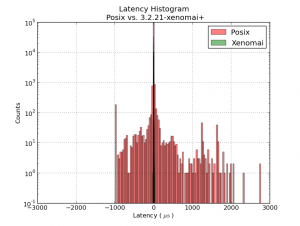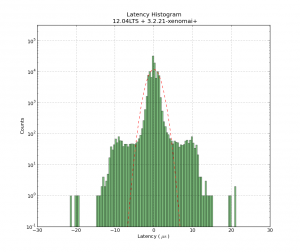## Why Real-Time?

Why bother with these real-time kernels and APIs at all? Isn't timing on a modern PC good enough? Look at this:This histogram shows latency-numbers from the same 1ms thread test run compiled without (red) and with (green) real-time enabled. All the green real-time data clusters around zero +/- 20us. Without real-time enabled the event we are expecting to happen every 1 ms might happen almost 1 ms too early, or up to 3 ms late. With real-time the timing is mostly consistent to better than 1% (10 us) with a worst-case jitter of 2% (20 us).

## Latency HistogramThis shows a latency-histogram for a 1 ms thread running on Xenomai on my recently acquired ITX-board. Note how badly the histogram is approximated by a normal distribution (Gaussians look like parabolas with logarithmic y-scale!). See also Michael's recent RPi data and  Kent's Athlon/P4 data.

The usual latency-test numbers people report is the maximum latency, a measure of how far out to the left or right the most distant single data point lies. The histrogram can probably be used to extract many more numbers, but for real-time critical applications like cnc-machine control the maximum latency is probably an OK figure of merit.

The latency numbers are recorded with a simple HAL component:lhisto.comp

The instantaneous latency-number is then put in a FIFO by the real-time component sampler and written to a text-file using halsampler. I'm setting this up with the following HAL commands (put this in a file myfile.halrun and run with "halrun -f myfile.halrun")

1 2 3 4 5 6 7 8 9 10 11 12 13 14 15 16 17 18 19 20 21 22 23 24 import numpy as np import matplotlib.pyplot as plt import matplotlib.mlab as mlab # load data from file x = np.loadtxt('latencysamples.txt' ) x=x/1e3 # convert to microseconds   fig = plt.figure() ax = fig.add_subplot(111) nbins = len(x)/1000 n, bins, patches = ax.hist(x, nbins, facecolor='green', alpha=0.5, log=True) bincenters = 0.5*(bins[1:]+bins[:-1]) # from matlplotlib example code mu = np.mean(x) sigma = np.std(x) area = np.trapz(n,bincenters) # scale normpdf to have the same area as the dataset y = area * mlab.normpdf( bincenters, mu, sigma) l = ax.plot(bincenters, y, 'r--', linewidth=1)# add a 'best fit' line for the normal PDF   ax.set_xlabel('Latency ( $\mathrm{ \mu s }$ ) ') ax.set_ylabel('Counts') ax.set_title('Latency Histogram\n 12.04LTS + 3.2.21-xenomai+') ax.set_ylim(1e-1, 10*max(n)) ax.grid(True) plt.show()